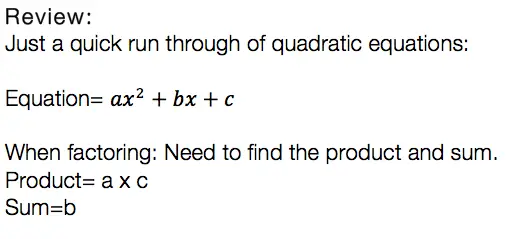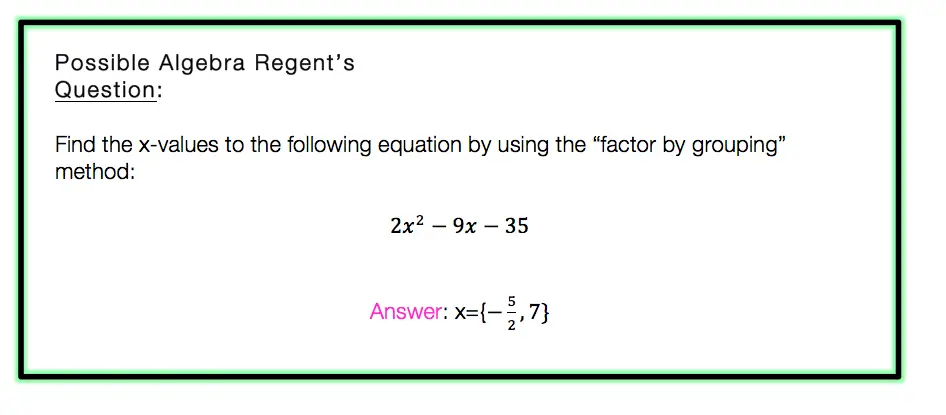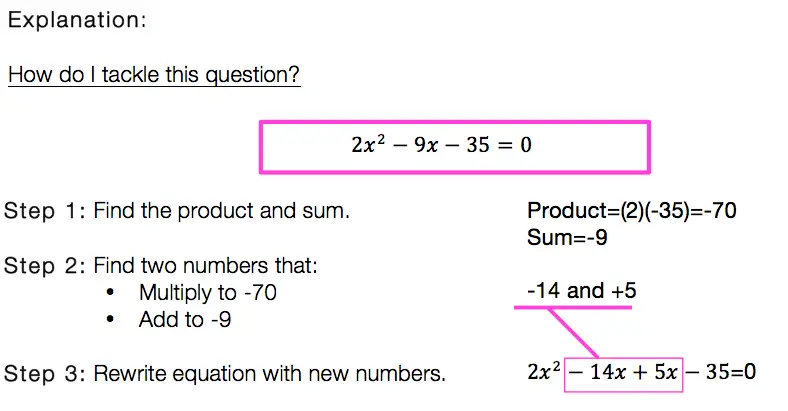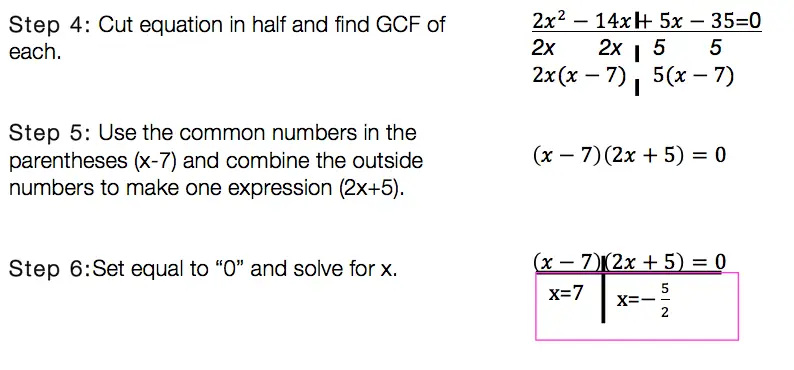# Factor by Grouping: Algebra 2/Trig.

Hey math friends! In this post, we are going to go over Factor by Grouping, one of the many methods for factoring a quadratic equation.  There are so many methods to factor quadratic equations, but this is a great choice, for when a is greater than 1. Also, if you need to review different types of factoring methods, just check out this link here.  Stay curious and happy calculating! 🙂 Before we go any further, let’s just take a quick look at what a quadratic equation is:Usually, we can just find the products, the sum, re-write the equation, solve for x, and be on our merry way.  But if you notice, there is something special about the question below. The coefficient “a” is greater than 1/. This is where factor by grouping comes in handy!Now that we know why and when we need to factor by grouping lets take a look at our Example:Factor By Grouping: Hard to solve? No.  Hard to remember? It can be, just remember to practice, practice practice! Also, if you are in need of a review of other methods of factoring quadratic equations, click this link here.

Want more Mathsux?  Don’t forget to check out our Youtube channel and more below! And if you have any questions, please don’t hesitate to comment. Happy Calculating! 🙂

Looking for more on Quadratic Equations and Functions? Check out the following Related posts!

Factoring Review

What is the Discriminant?

Completing the Square

4 Ways to Factor a Trinomial

Is it a Function?

Imaginary and Complex Numbers

Quadratic Equations with 2 Imaginary Solutions

Focus and Directrix of a Parabola